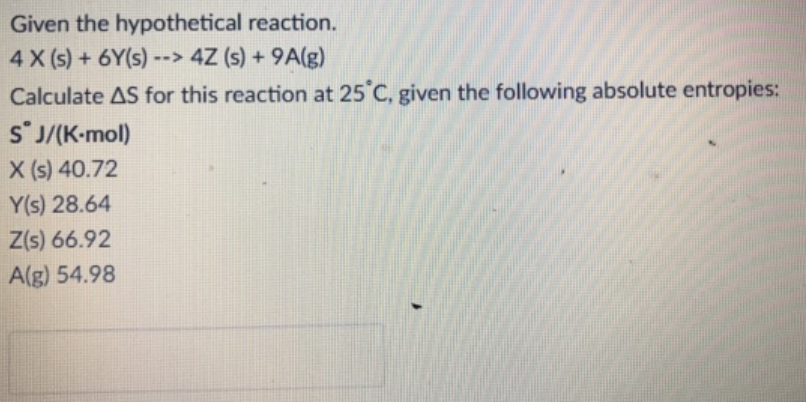# Problem: Given the hypothetical reaction. 4 X(s) + 6Y(s) → 4Z (s) + 9A(g) Calculate Δ S for this reaction at 25°C, given the following absolute entropies: S°J/(K-mol) X (s) 40.72 Y(s) 28.64 Z(s) 66.92 A(g) 54.98

###### FREE Expert Solution###### Problem Details
Given the hypothetical reaction.
4 X(s) + 6Y(s) → 4Z (s) + 9A(g)
Calculate Δ S for this reaction at 25°C, given the following absolute entropies:
S°J/(K-mol)
X (s) 40.72
Y(s) 28.64
Z(s) 66.92
A(g) 54.98